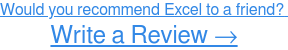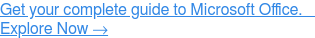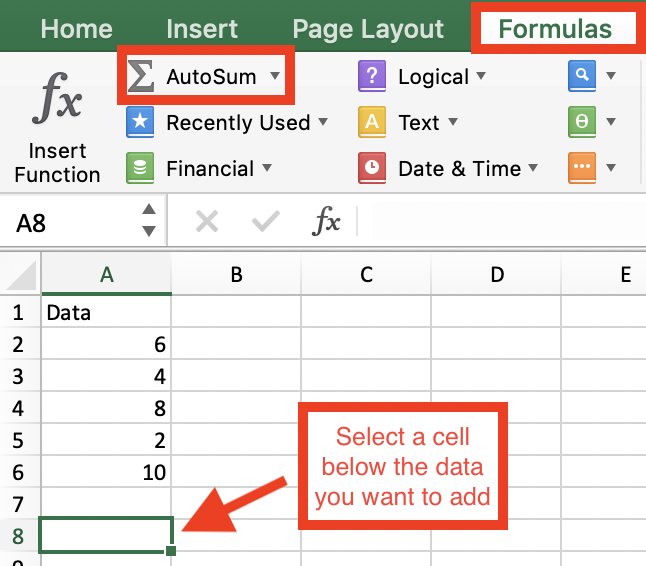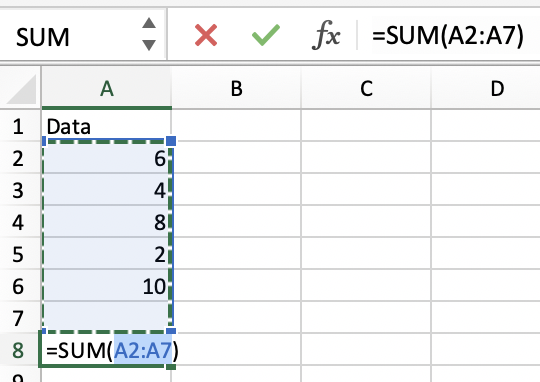Microsoft Excel is a great tool for basic math operations.

And while I’ve previously covered how to subtract in Excelhow to multiply in Excel, and how to divide in Excel, this article will cover how to add in Excel – the following to be specific:If you’re looking to add in Excel, you’ll need to know how to write Excel formulas.

Excel formulas are expressions used to display values that are calculated from the data you’ve entered and they’re generally used to perform various mathematical, statistical, and logical operations.

So before we jump into the specifics of how to add in Excel, here are a few reminders to keep in mind when writing Excel formulas:

• All Excel formulas should begin with the equal sign (=). This is a must in order for Excel to recognize it as a formula.
• The cells will display the result of the formula, not the actual formula. So by using cell references rather than just entering the data in the cell, if you need to go back later the values will be easier to understand.
• When using arithmetic operators in your formula, remember the order of operations (I personally reference the acronym PEMDAS: Parenthesis, Exponentiation, Multiplication, Division, Addition, Subtraction.) Below you’ll find an example of how the order of operations is applied to formulas in Excel.## How to add numbers in Excel

To add numbers in a cell, you'll need to write a formula in the designated cell. Your formula should start with the equal sign (=), and contain the arithmetic operator needed for the calculation. In this case, you want to add 12 to 6, so your formula is =12+6.Once you’ve entered the formula, press ‘Enter’ and your result will populate. In this example, the addition formula, =12+6, yields a result of 18 (see below).## How to add cells in Excel

To add cell references, your data will need to be in separate cells.

You can see below that an addition formula was written in cell B2 using cell references, but the data had to be entered separately in cells A2 and A3.After writing your formula, press ‘Enter’ and your result will populate. In this case, =A2+A3 yields 18.## How to use the SUM function in Excel

The SUM function is used to add values – individual values, cell references, and ranges of cells. Below I’ll walk through an example of each.

To start, navigate to the ‘Formulas’ tab and click ‘Insert Function.’The Formula Builder should pop up on the right side of your screen. Here you can search for the SUM function if it’s not already on your main menu. Click ‘SUM’ and ‘Insert Function.’From here, enter the individual values that you want to add. Press the ‘+’ sign to add more numbers if needed. Your SUM formula will build in the cell as you add values.Once you’ve added the values, click ‘Done’ or press ‘Enter’ and the sum of the individual values will populate. In this case, 8+2 results in 10.Similar to adding cell references without the SUM function, data must be entered in separate cells for this calculation.

Start by selecting the cell you want the result to populate in and type ‘=SUM.’ Select ‘SUM’ in the drop-down menu.From here, enter the first cell reference (A2) followed by a comma (,) and the second cell reference (A3).

Note: You can add more than two cell references – just remember that each cell reference must be followed by a comma.Press ‘Enter’ and the sum of the cell references will populate. In this case, the sum of cells A2 and A3 is 8.### Adding a range of cells

Just as you would when adding cell references, start by selecting the cell you want the result to populate in and type ‘=SUM.’ Select ‘SUM’ in the drop-down menu.Click the first cell in the range of cells you want to add together.Drag the cursor to highlight the full range of cells.Press ‘Enter’ and the sum of the cell range will populate.## How to use AutoSum in Excel

AutoSum is very similar to the SUM function – the exact same, actually – except Excel writes the formula for you here!

Trying to figure out how to add columns in Excel? AutoSum is a good start.

To use AutoSum, navigate to the ‘Formulas’ tab, select a cell below the last value in the column (or row) that you want to add, and click the ‘AutoSum’ button.

##Excel will automatically highlight the range of cells and enter the formula (using the SUM function) to sum the numbers.Adjust the formula if needed (Excel is smart, so you probably won’t have to), and press ‘Enter.’

And just like that Excel did the math for you! Pretty cool, huh?There you have it! In this article, we’ve covered everything you need to know about how to add in Excel using formulas and functions.

Now grab your computer and dive into Excel to give it a try for yourself!

Did you enjoy this article? Check out our articles on how merge cells in Excel and how to lock cells in Excel!

Stay tuned for more articles on Microsoft Excel soon!

# Never miss a post.

Subscribe to keep your fingers on the tech pulse.